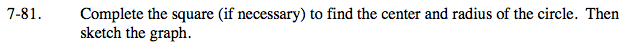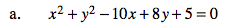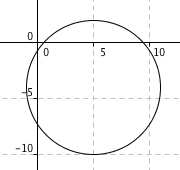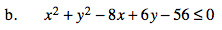### Home > PC > Chapter 7 > Lesson 7.2.4 > Problem7-81

7-81.Regroup with x's and y's together and the constant on the other side.

(x2 − 10x + ?) + (y2 + 8y + ?) = − 5

Complete the square finding the ?'s and adding the same amount on both sides.

(x2 − 10x + 25) + (y2 + 8y + 16) = − 5 + 25 + 16

Simplify and graph.

(x − 5)2 + (y + 4)2 = 36Follow the same thinking process as (a) above.